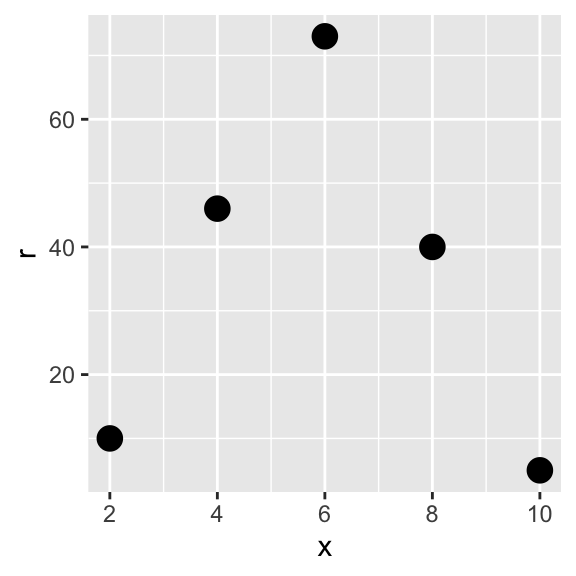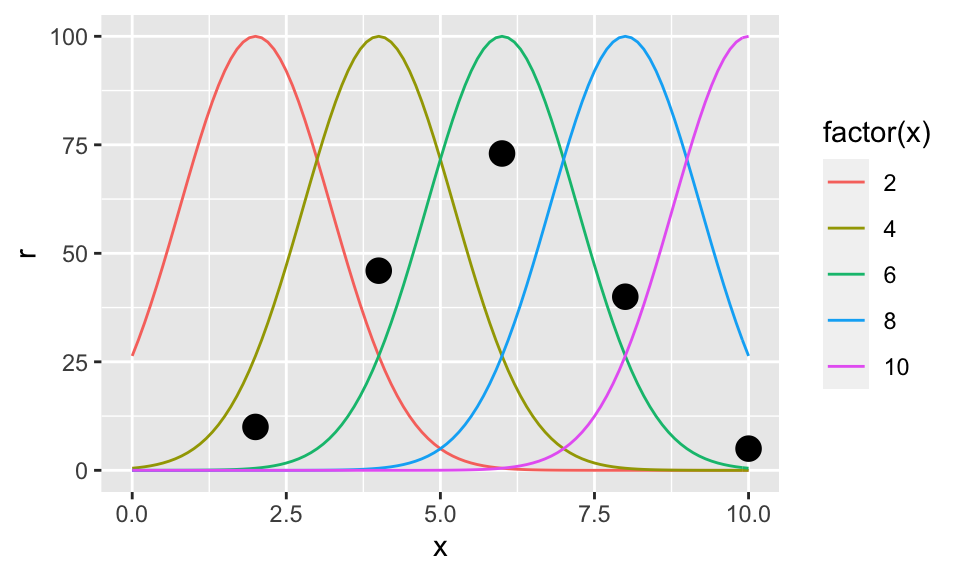In the simpler case of MLE, we have some iid (independent and identically distributed) observations and we want to estimate from the parametric family of distributions, which is the most likely distribution. In neuroscience often we have some observations, the response of some neurons, $$r=(r_1, r_2,..r_n)$$ that are independent but no identically distributed because they are indexed by another variable $$x_1,x_2,...,x_n$$. For example, $$r_i$$ could be the number of spikes of a neuron that responds maximally when the stimulus is $$x_i$$. We need to use a generalization of the likelihood function that includes the index $L(\theta|x,r)=p(x,r|\theta)$

where p is the joint density function (I replaced $$f$$ by $$p$$ because it is more common in neuroscience) Suppose that we have the following independent responses for neurons that are tuned for different speeds (respond maximally to different speeds)

r<-c(10,46,73,40,5)
x<-c(2,4,6,8,10) # speeds in deg/s

Let’s plot the responses as a function of the tuning speed

library('ggplot2')
dat<-data.frame(x,r) #we plotted our observations in the x-axis
p<-ggplot(data=dat,aes(x=x,y=r))+geom_point(size=4)
pTo decode $$\theta$$ from the experiment, we need to extract $$p(x,r\mid\theta)$$ which can be difficult (to decode $$\theta$$ using a vector method, for example, only requires the knowledge of the preferred values $$x_i$$). Let’s consider a simple situation in which we don’t know the exact probability density functions for $$r_1, r_2...r_n$$, but we know that the shape is the shape of the poison distribution where $$\lambda$$ (the mean response) is indexed by x following the tuning function f that depends on the paramater $$\theta$$ that we want to estimate $\lambda=f(x,\theta).$ Then, the probability density function for $$r_i$$ is $p(x_i,r_i\mid\theta)=p(x_i,r_i\mid \lambda)=p(r_i\mid f(x_i,\theta))=\frac{f(x_i,\theta)^{r_i}}{r_i!}e^{-f(x_i,\theta)}$

Let’s supppose that $$r_i$$ are independent, the join density function is the multiplication of the probability density function for each $$r_i$$ $p(r\mid \theta)=p(r_1,r_2...r_n\mid \theta)=p(x_1,r_1\mid \theta)p(x_2,r_2\mid \theta)...p(x_n,r_n\mid \theta)=$ $=\prod_{i=1}^{n}\frac{f(x_i,\theta)^{r_i}}{r_i!}e^{-f(x_i,\theta)}$

that when considered as a function of the parameter $$\theta$$ is the likelihood function $L(\theta\mid r)=L(\theta\mid r).$

The log likelihood is $log(L(\theta\mid r))=\sum_{i=1}^{n}\left\{ r_ilog(f(x_i,\theta))-log(r_i!)-f(x_i,\theta)\right\}$

To calculate $$\theta$$ that maximizes the log likelihood the second term can be ignored as does not depend on $$\theta$$. The third can be ignored when the tuning curves are dense and evenly distributed because the sum is approximately independent of $$\theta$$ (Dayan & Abbott, 2001).

Let’s suppose that the tuning functions are gaussians distributions with standard deviation 3 and maximum firing rate 100

library('plyr')
f<-function(p,x) { #p is theta
a<-100; sigma<-3
a*exp(-(p-x)^2/sigma) #tuning curve of a neuron center on x
}
xSeq<-seq(0,10,.1)
fs<-ddply(data.frame(x),.(x),function(d){
ySeq<-f(xSeq,d$x) data.frame(xSeq,ySeq) }) p<-p+geom_line(data=fs,aes(x=xSeq,y=ySeq,color=factor(x))) pLet’s calculate the log likelihood for a couple of values of the parameter $$\theta$$ logL<-function(p) sum( r*log(f(p,x))-f(p,x) ) logL(6) ##  452.9479 logL(3) ##  0.3239317 logL(6)>logL(3) ##  TRUE Given the responses r, it is much more likely that the speed is 6 than 3. Let’s calculate it properly optimize(logL,c(0,19),maximum=T)$maximum
##  5.81327

Given that the tuning functions are gaussians with the same width and assuming that the third term in the log likelihood above is small, it could be demonstrated that the maximum likelihood estimator is (Dayan & Abbott, 2001) $\theta_0=\frac{\sum_{i=1}^{n}r_i x_i}{\sum_{i=1}^{n}r_i}$

sum(r*x)/sum(r)
##  5.816092